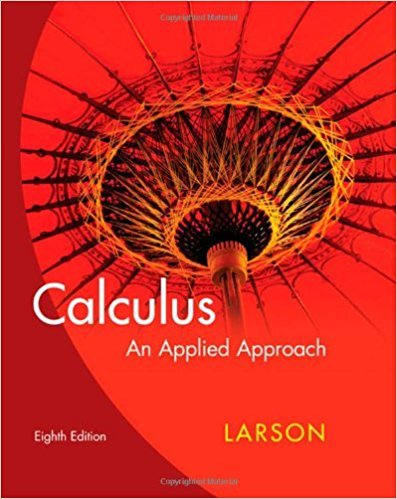×
×

# Solutions for Chapter 6.1: Integration by Parts and Present Value## Full solutions for Calculus: An Applied Approach | 8th Edition

ISBN: 9780618958252Solutions for Chapter 6.1: Integration by Parts and Present Value

Solutions for Chapter 6.1
4 5 0 287 Reviews
19
0
##### ISBN: 9780618958252

This expansive textbook survival guide covers the following chapters and their solutions. This textbook survival guide was created for the textbook: Calculus: An Applied Approach , edition: 8. Calculus: An Applied Approach was written by and is associated to the ISBN: 9780618958252. Since 82 problems in chapter 6.1: Integration by Parts and Present Value have been answered, more than 21900 students have viewed full step-by-step solutions from this chapter. Chapter 6.1: Integration by Parts and Present Value includes 82 full step-by-step solutions.

Key Calculus Terms and definitions covered in this textbook
• Absolute value of a vector

See Magnitude of a vector.

• Additive inverse of a real number

The opposite of b , or -b

• Binomial coefficients

The numbers in Pascal’s triangle: nCr = anrb = n!r!1n - r2!

• Common difference

See Arithmetic sequence.

• Compound fraction

A fractional expression in which the numerator or denominator may contain fractions

• Explanatory variable

A variable that affects a response variable.

• Explicitly defined sequence

A sequence in which the kth term is given as a function of k.

• Extracting square roots

A method for solving equations in the form x 2 = k.

• Fundamental

Theorem of Algebra A polynomial function of degree has n complex zeros (counting multiplicity).

• Initial point

See Arrow.

• Least-squares line

See Linear regression line.

• Multiplicative inverse of a matrix

See Inverse of a matrix

• NINT (ƒ(x), x, a, b)

A calculator approximation to ?ab ƒ(x)dx

• Period

See Periodic function.

• Polar coordinate system

A coordinate system whose ordered pair is based on the directed distance from a central point (the pole) and the angle measured from a ray from the pole (the polar axis)

• Probability of an event in a finite sample space of equally likely outcomes

The number of outcomes in the event divided by the number of outcomes in the sample space.

• Rational numbers

Numbers that can be written as a/b, where a and b are integers, and b ? 0.

• Reference angle

See Reference triangle

• Richter scale

A logarithmic scale used in measuring the intensity of an earthquake.

• Stretch of factor c

A transformation of a graph obtained by multiplying all the x-coordinates (horizontal stretch) by the constant 1/c, or all of the y-coordinates (vertical stretch) of the points by a constant c, c, > 1.

×